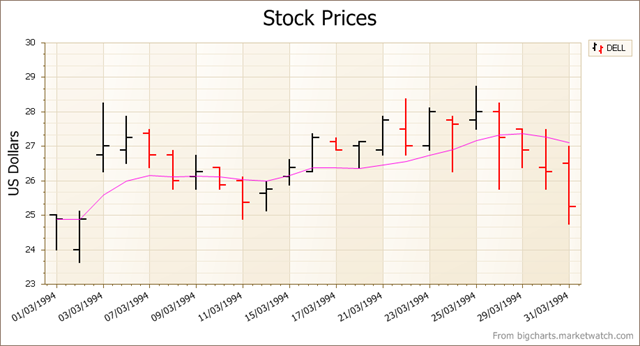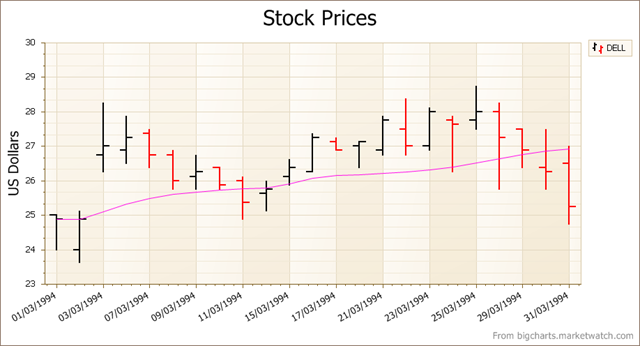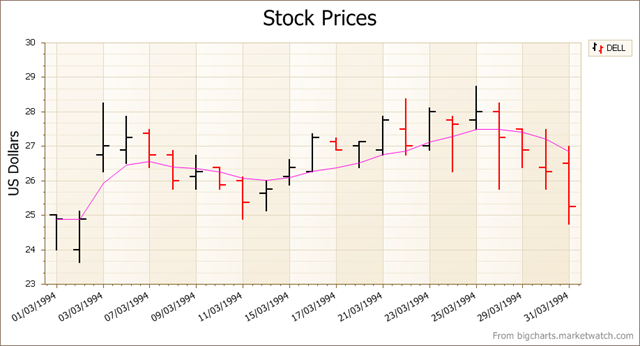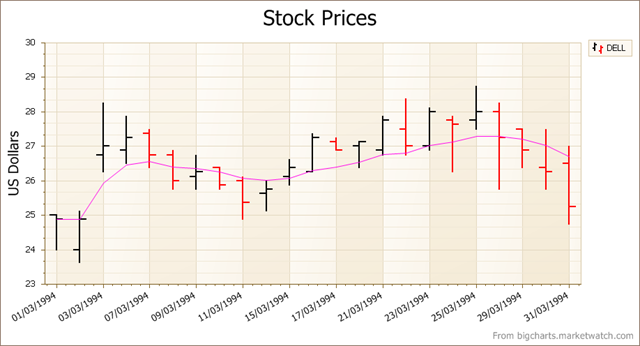# WinForms and ASP.NET Charting: moving averages (coming in v2010.2)

One of the pleasures of being in a downtime at a show like DevConnections is that you can browse the What’s New of our various products through the new Demo Center. Yes, I know I get notified of the new features well in time, but there’s nothing like actually seeing the feature in action.

One nice example presented itself 10 minutes ago or so as I was playing with the demos for XtraCharts on WinForms. We’re continuing to add trend lines to our financial charts to make that whole sub-genre of charts more valuable for our customers and I came across the Moving Averages demo.

Here’s the simple moving average (SMA) trend line for a set of stock prices for Dell:As its name suggests: a simple moving average. The chart calculates the average price over a period of time (here, 10 days) for each date. So for day 10, the average for days 1 through 10 is calculated, for day 11 you use days 2 to 11, and so on. Pretty easy.

Next up is the triangular moving average (TMA). If you describe the SMA as a smoothing operation on the prices so that can more easily see overall trends (rather than the spiky day by day prices), you could describe a TMA as a double smoothing operation. Here the chart calculates the simple moving average of all the SMAs previously calculated. (For day 10 then, the chart calculates the average of the SMAs on days 1 through 10). This double smoothing operation seems to help show deeper changes in direction of the price than does the SMA.

Here’s the TMA for the same data:Now we can consider the weighted moving average (WMA). Here the prices that are more recent have more influence (are weighted more) than those earlier on. The weighting used is simple: given a period of 10 days for calculating the average, the most recent price is given a weighting of 10, the previous one a weighting of 9, and so on. Of course, the divisor for the average becomes the sum of the weights.

Here’s the WMA for the same data.We can then move to the exponential moving average (EMA). This uses a weighting factor that again prizes more recent prices over earlier ones, but this one decreases the weights exponentially rather than arithmetically.

Here’s the EMA for the same data:For trading type applications, these and the other financial trend lines provided in XtraCharts give you a much better analytic environment to study stock price trends.

(Oh, and before you ask, these new moving average trend lines are fully supported in ASP.NET as well.)

Tags
3 comment(s)Bruno Cossi

Very useful! Well done.

4 November, 2010Bruno Cossi

Very useful! Well done.

4 November, 2010thanhtung nguyen

very good,

5 November, 2010